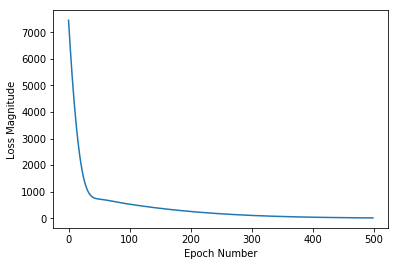!pip install tensorflow

Requirement already satisfied: tensorflow in /srv/paws/lib/python3.6/site-packages
Requirement already satisfied: six>=1.10.0 in /srv/paws/lib/python3.6/site-packages (from tensorflow)
Requirement already satisfied: wheel>=0.26 in /srv/paws/lib/python3.6/site-packages (from tensorflow)
Requirement already satisfied: keras-applications>=1.0.6 in /srv/paws/lib/python3.6/site-packages (from tensorflow)
Requirement already satisfied: grpcio>=1.8.6 in /srv/paws/lib/python3.6/site-packages (from tensorflow)
Requirement already satisfied: tensorboard<1.14.0,>=1.13.0 in /srv/paws/lib/python3.6/site-packages (from tensorflow)
Requirement already satisfied: numpy>=1.13.3 in /srv/paws/lib/python3.6/site-packages (from tensorflow)
Requirement already satisfied: termcolor>=1.1.0 in /srv/paws/lib/python3.6/site-packages (from tensorflow)
Requirement already satisfied: protobuf>=3.6.1 in /srv/paws/lib/python3.6/site-packages (from tensorflow)
Requirement already satisfied: keras-preprocessing>=1.0.5 in /srv/paws/lib/python3.6/site-packages (from tensorflow)
Requirement already satisfied: gast>=0.2.0 in /srv/paws/lib/python3.6/site-packages (from tensorflow)
Requirement already satisfied: astor>=0.6.0 in /srv/paws/lib/python3.6/site-packages (from tensorflow)
Requirement already satisfied: tensorflow-estimator<1.14.0rc0,>=1.13.0 in /srv/paws/lib/python3.6/site-packages (from tensorflow)
Requirement already satisfied: absl-py>=0.1.6 in /srv/paws/lib/python3.6/site-packages (from tensorflow)
Requirement already satisfied: h5py in /srv/paws/lib/python3.6/site-packages (from keras-applications>=1.0.6->tensorflow)
Requirement already satisfied: werkzeug>=0.11.15 in /srv/paws/lib/python3.6/site-packages (from tensorboard<1.14.0,>=1.13.0->tensorflow)
Requirement already satisfied: markdown>=2.6.8 in /srv/paws/lib/python3.6/site-packages (from tensorboard<1.14.0,>=1.13.0->tensorflow)
Requirement already satisfied: setuptools in /srv/paws/lib/python3.6/site-packages (from protobuf>=3.6.1->tensorflow)
Requirement already satisfied: mock>=2.0.0 in /srv/paws/lib/python3.6/site-packages (from tensorflow-estimator<1.14.0rc0,>=1.13.0->tensorflow)
Requirement already satisfied: pbr>=0.11 in /srv/paws/lib/python3.6/site-packages (from mock>=2.0.0->tensorflow-estimator<1.14.0rc0,>=1.13.0->tensorflow)

from __future__ import absolute_import, division, print_function
import tensorflow as tf

tf.logging.set_verbosity(tf.logging.ERROR)
import numpy as np

celsius_q    = np.array([-40, -10,  0,  8, 15, 22,  38],  dtype=float)
fahrenheit_a = np.array([-40,  14, 32, 46, 59, 72, 100],  dtype=float)

# f = 𝑐×1.8+32
for i,c in enumerate(celsius_q):
print("{} degrees Celsius = {} degrees Fahrenheit".format(c, fahrenheit_a[i]))

-40.0 degrees Celsius = -40.0 degrees Fahrenheit
-10.0 degrees Celsius = 14.0 degrees Fahrenheit
0.0 degrees Celsius = 32.0 degrees Fahrenheit
8.0 degrees Celsius = 46.0 degrees Fahrenheit
15.0 degrees Celsius = 59.0 degrees Fahrenheit
22.0 degrees Celsius = 72.0 degrees Fahrenheit
38.0 degrees Celsius = 100.0 degrees Fahrenheit

"""
input_shape= — This specifies that the input to this layer is a single value.
That is, the shape is a one-dimensional array with one member.
Since this is the first (and only) layer, that input shape is the
input shape of the entire model. The single value is a floating point number,
representing degrees Celsius.

units=1 — This specifies the number of neurons in the layer.
The number of neurons defines how many internal variables the layer has to try to learn how
to solve the problem (more later). Since this is the final layer,
it is also the size of the model's output — a single float value representing degrees
Fahrenheit. (In a multi-layered network, the size and shape of the layer would
need to match the input_shape of the next layer.)
"""
# no activation function this means activation is linear
layer0 = tf.keras.layers.Dense(units=1, input_shape=)

"""
Once layers are defined, they need to be assembled into a model.
The Sequential model definition takes a list of layers as argument,
specifying the calculation order from the input to the output.

This model has just a single layer, l0.

"""
model = tf.keras.Sequential([layer0])

"""
Before training, the model has to be compiled. When compiled for training, the model is given:

Loss function — A way of measuring how far off predictions are from the desired outcome.
(The measured difference is called the "loss".

Optimizer function — A way of adjusting internal values in order to reduce the loss.
"""
model.compile(loss='mean_squared_error',

history = model.fit(celsius_q, fahrenheit_a, epochs=500, verbose=False)
print("Finished training the model")

Finished training the model

import matplotlib.pyplot as plt
plt.xlabel('Epoch Number')
plt.ylabel("Loss Magnitude")
plt.plot(history.history['loss'])

[<matplotlib.lines.Line2D at 0x7f6c6883f898>]print(model.predict([100.0]))

[[211.2817]]

print ("weights {}". format(layer0.get_weights()))

weights [array([[1.8282561]], dtype=float32), array([28.456083], dtype=float32)]

print (layer0)

<tensorflow.python.keras.layers.core.Dense object at 0x7f6c68ed4f28>

'''
Now pretednd this is real life and we do not know how many layers thi smodel should have
'''

layer0 = tf.keras.layers.Dense(units=4, input_shape=)
layer1 = tf.keras.layers.Dense(units=4)
layer2 = tf.keras.layers.Dense(units=1)

model = tf.keras.Sequential([layer0, layer1, layer2])

model.compile(loss='mean_squared_error',

history = model.fit(celsius_q, fahrenheit_a, epochs=500, verbose=False)
print(model.predict([100.0]))

[[211.74744]]

print("These are the l0 variables: {}".format(layer0.get_weights()))
print("These are the l1 variables: {}".format(layer1.get_weights()))
print("These are the l2 variables: {}".format(layer2.get_weights()))

These are the l0 variables: [array([[-0.4106331 , -0.28890714, -0.46830842,  0.16782592]],
dtype=float32), array([-3.22501  , -3.500317 , -2.7259123, -1.9343193], dtype=float32)]
These are the l1 variables: [array([[ 0.21468374,  0.8340269 ,  0.6283667 ,  0.63672704],
[-0.8861123 , -0.00929915,  1.493446  ,  1.0691962 ],
[ 0.8034012 , -0.13222514,  0.38455197,  0.49358302],
[-0.07090295,  1.4334207 , -0.06197542,  0.15114348]],
dtype=float32), array([-2.4430873, -2.7279053, -3.5594342, -3.7882783], dtype=float32)]
These are the l2 variables: [array([[-0.14975283],
[-0.54845077],
[-1.2674643 ],
[-0.81061083]], dtype=float32), array([3.3844879], dtype=float32)]